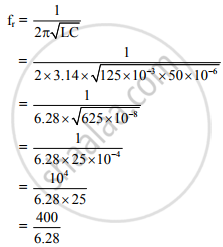HSC Science (General) 12th Board ExamMaharashtra State Board
Share

An A.C. Circuit Consists of Inductor of Inductance 125 Mh Connected in Parallel with a Capacitor of Capacity 50 μF. Determine the Resonant Frequency. - HSC Science (General) 12th Board Exam - Physics

Question

An A.C. circuit consists of inductor of inductance 125 mH connected in parallel with a capacitor of capacity 50 μF. Determine the resonant frequency.

Solution

L = 125 mH = 125 × 10−3H,
C = 50 µF = 50 × 10−6F
fr = ?
We have,= antilog [log (400) – log (6.28)]
= antilog [2.6021 – 0.7980]
= antilog [1.8041]
∴ fr = 63.69 Hz

The resonant frequency in the A.C. circuit is 63.69 Hz.

Is there an error in this question or solution?

APPEARS IN

2013-2014 (October) (with solutions)
Question 6.7 | 2.00 marks

Video TutorialsVIEW ALL 

Solution An A.C. Circuit Consists of Inductor of Inductance 125 Mh Connected in Parallel with a Capacitor of Capacity 50 μF. Determine the Resonant Frequency. Concept: Resonant Circuit.
S